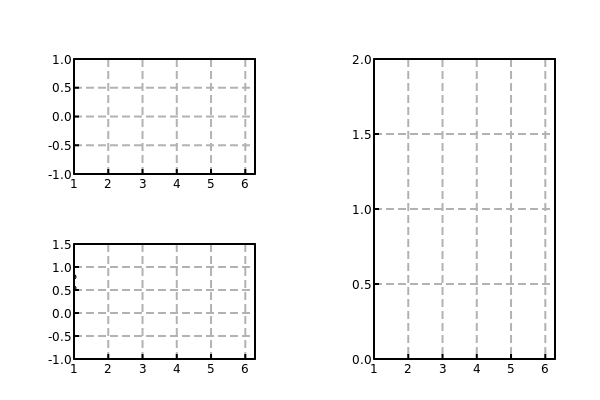# Animation with subplotsusing Plots
inspectdr()

The layout macro can be used to create an animation with subplots.

l = @layout([[a; b] c])
p = plot(plot([sin, cos], 1, ylims = (-1, 1), leg = false), scatter([atan, cos], 1, ylims = (-1, 1.5), leg = false), plot(log, 1, ylims = (0, 2), leg = false), layout = l, xlims = (1, 2π))
anim = Animation()
for x = range(1, stop = 2π, length = 20)
plot(push!(p, x, Float64[sin(x), cos(x), atan(x), cos(x), log(x)]))
frame(anim)
end

gif(anim, "assets/anim_inspectdr_ex31.gif")This page was generated using DemoCards.jl and Literate.jl.